# MySQL SUM() function

## SUM() function

MySQL SUM() function returns the sum of an expression. SUM() function returns NULL when the return set has no rows. It's a fundamental aggregate function that provides valuable insights into data analysis and numerical calculations.

This function is useful in -

• This is essential for understanding the cumulative value of a dataset.
• SUM() is used to aggregate data, making it easier to present the combined value of multiple records.
• For inventory tracking, the SUM() function calculates the total quantity of products in stock or sold.
• SUM() helps in validating calculations by comparing the expected sum with the calculated sum to identify discrepancies.
• In performance analysis, SUM() assists in evaluating cumulative metrics, such as total sales over a period.
• When generating summary reports, the SUM() function is employed to show the total value of specific attributes.
• In resource management, SUM() helps calculate the total utilization or consumption of resources.

Syntax:

```SUM([DISTINCT] expr)
```

Where expr is an expression.

The DISTINCT keyword can be used to sum only the distinct values of expr.

MySQL Version: 8.0

Example: MySQL SUM() function

The following MySQL statement returns the sum of 'total_cost' from purchase table.

Sample table: purchase

Code:

``````SELECT SUM(total_cost)
FROM purchase;
```
```

Relational Algebra Expression: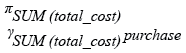Relational Algebra Tree: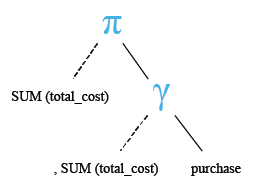Output:

```mysql> SELECT SUM(total_cost)
-> FROM purchase;
+-----------------+
| SUM(total_cost) |
+-----------------+
|         3590.00 |
+-----------------+
1 row in set (0.00 sec)
```

Example: MySQL SUM() function with where clause

MySQL SUM() function with WHERE retrieves the sum of a given expression which is filtered against a condition placed after WHERE. The following MySQL statement returns the sum of 'total_cost' from purchase table for the category ('cate_id') given with WHERE clause.

Sample table: purchase

Code:

``````SELECT SUM(total_cost)
FROM purchase
WHERE cate_id='CA001';
```
```

Relational Algebra Expression: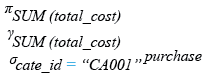Relational Algebra Tree: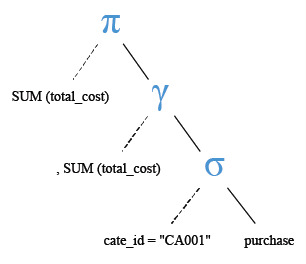Output:

```mysql> SELECT SUM(total_cost)
-> FROM purchase
-> WHERE cate_id='CA001';
+-----------------+
| SUM(total_cost) |
+-----------------+
|         1725.00 |
+-----------------+
1 row in set (0.00 sec)
```

Example: MySQL SUM() function using multiple columns

MySQL SUM() function retrieves the sum value of an expression which is made up of more than one columns. The above MySQL statement returns the sum of multiplication of 'receive_qty' and 'purch_price' from purchase table for each group of category ('cate_id') .

Sample table: purchase

Code:

``````SELECT cate_id,
FROM purchase
GROUP BY cate_id;
```
```

Relational Algebra Expression: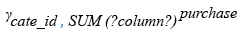Relational Algebra Tree: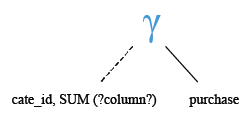Output:

```mysql> SELECT cate_id,
-> FROM purchase
-> GROUP BY cate_id;
+---------+------------------------------+
+---------+------------------------------+
| CA001   |                      1725.00 |
| CA002   |                       965.00 |
| CA003   |                       900.00 |
+---------+------------------------------+
3 rows in set (0.02 sec)
```

Example: MySQL SUM() function with COUNT() function and variables

The following MySQL statement will return the sum of the ‘mysum’, a temporary variable which counts number of books containing more than 200 pages from 'book_mast' table.

Sample table: book_mast

Code:

``````SELECT SUM(mysum)
FROM(
SELECT COUNT(*) AS  mysum
FROM book_mast
WHERE no_page>200) AS bb;
```
```

Relational Algebra Expression: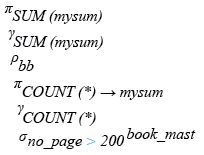Relational Algebra Tree: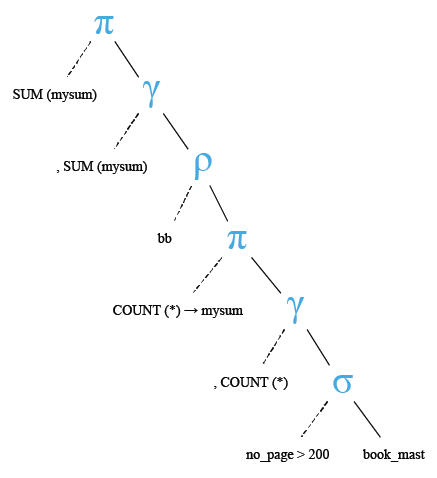Output:

```mysql> SELECT SUM(mysum)
-> FROM(
-> SELECT COUNT(*) AS  mysum
-> FROM book_mast
-> WHERE no_page>200) AS bb;
+------------+
| SUM(mysum) |
+------------+
|         12 |
+------------+
1 row in set (0.02 sec)
```

Example: MySQL SUM() function with DISTINCT clause

MySQL SUM() function retrieves the sum of a unique value of an expression if it is accompanied by DISTINCT clause. The following MySQL statement returns the sum of a number of branches ('no_of_branch') from publisher table, where, if more than one publisher has the same number of branches, that number (i.e. number of branches) is taken once only.

Sample table: publisher

Code:

``````SELECT SUM(DISTINCT no_of_branch)
FROM publisher;
```
```

Relational Algebra Expression: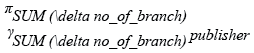Relational Algebra Tree: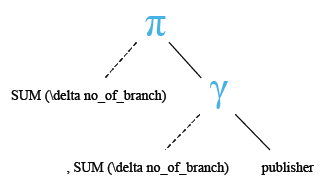Output:

```mysql> SELECT SUM(DISTINCT no_of_branch)
-> FROM publisher;
+----------------------------+
| SUM(DISTINCT no_of_branch) |
+----------------------------+
|                         64 |
+----------------------------+
1 row in set (0.00 sec)
```

Previous: STDDEV()
Next: SUM() with group by

﻿